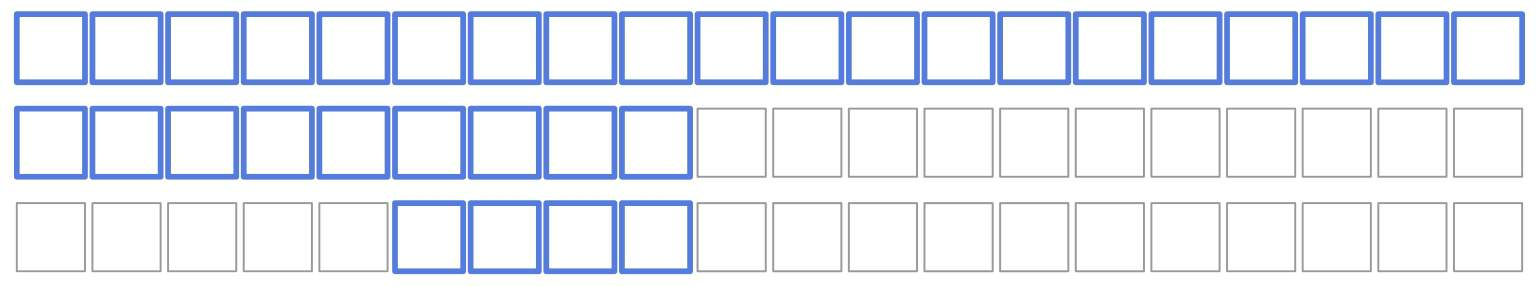## 1. Introduction

In this tutorial, we’ll derive the complexity of binary search.

Binary search is an efficient algorithm for finding the position of an item in a sorted array.

So, in this problem, we have a sorted array(such that) and a search key. We want to find ifis inand at what position.

The assumption is the input array is sorted, which requires its elements to be comparable. So, there has to be an ordering relation on the class of elements from which we drawand.

## 3. The Algorithm

Binary search comparesto the middle elementof the array and focuses on the left or right subarrays depending on whetheror. If, we output:

Here’s the pseudocode:So, the search stops when there are no more elements to search or we find the search key.

## 4. Complexity

Let’s callthe active part of the array. Since, we halve the active part in each iteration:In the worst case,isn’t in the array, so the number of iterations is:

•iterations to get to a one-element sub-array with• One additional iteration to break the loop

We do anwork in each iteration by comparingto the middle elements. So, the algorithm is logarithmic:.

### 4.1. Master Theorem

We can use the Master Theorem to find the complexity. Letbe the number of comparisons we perform in the worst case if the input array haselements.

Since we halve the active part and do at most two comparisons in each iteration, the recurrence is:The theorem uses the generic form:and comparesto. In our case,and, so, and.

Therefore, it holds that:From the Master Theorem, we get the following:### 4.2. Space Complexity

The binary search uses only a constant number of helper variables (). As a result, its iterative version (which we presented) has anauxiliary space complexity.

However, the recursive version would use astack memory and would be prone to the stack overflow error ifis large.

Since we needmemory for an array withelements, the total space complexity is.

## 5. Conclusion

In this article, we explained the complexity of binary search. The algorithm runs intime but works only for sorted arrays.